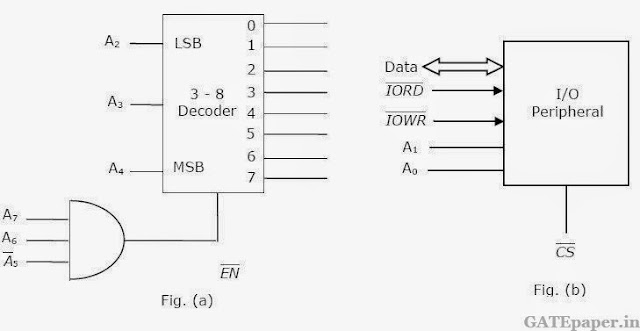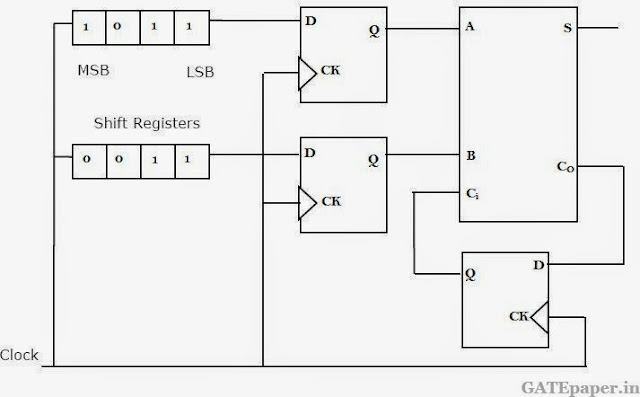### GATE 2006 ECE Video Solution on Digital Circuits (Digital Electronics)

1. The number of product terms in the minimized sum of product expression obtained through the following K-map (where “d” denotes don’t care states)
 1 0 0 1 0 d 0 0 0 0 d 1 1 0 0 1
a. 2
b. 3
c. 4
d. 5

2. A new Binary Coded Pentary (BCP) number system is proposed in which every digit of a base-5 number is represented by its corresponding 3-bit binary code. For example, the base-5 number 24 will be represented by its BCP code 010100. In this number system, the BCP code 100010011001 corresponds to the following number in base-5 system
a. 423
b. 1324
c. 2201
d. 4231

3. An I/O peripheral device shown n figure (b) below is to be interfaced to an 8085 microprocessor. To select the I/O device in the address range D4H – D7H, its chip-select (CS’) should be connected to the output of the decoder shown in the figure (a) below :a. Output 7
b. Output 5
c. Output 2
d. Output 0

4. For the circuit shown, two 4-bit parallel in serial out shift registers loaded with the data shown are used to fed the data to a full adder. Initially, all the flip-flops are in clear state. After applying two clock pulses, the outputs sum and carry of the full adder should be …….respectively.a. 0, 0
b. 0, 1
c. 1, 0
d. 1, 1

5. A 4 – bit D/A converter is connected to a free running 3 – bit UP counter, as shown in the following figure. Which of the following waveforms will be observed at Vo?6. Two D flip-flops are to be connected as a synchronous counter as shown below, that goes through the following Q1Q0 sequence 00 -> 01 -> 11 ->10 -> 00 ->………..
The inputs D0 and D1 respectively should be connected as7. Following is the segment of a 8085 assembly language program
LXI SP, EFFFH
CALL 3000H
:
:
3000H : LXI H, 3CF4H
PUSH PSW
SPHL
POP PSW
RET
On the completion of RET instruction, the contents of SP is
a. 3CF0H
b. 3CF8H
c. EFFDH
d. EFFFH
Solution :

8. The point P in the following figure is stuck-at-1. The output f will be